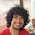### Invert the Cups

Source: Techfest 2010 Puzzle Hut

Problem: It is desired to invert a set of n upright cups by a series of moves in each of which n-1 cups are turned over. Show that this can always be done if n is even  and never can be done if n is odd.

Update(08/02/10):
Solution: Posted by Ankush Agarwal (2nd yr, CSE, IITB), Dinesh Dharme (4th yr, CSE, IITB), Nikhil (2nd yr, IITD) and Siddhant Agarwal (3rd year, EE, IITB) in comments!! For just solution, read Ankush's and Sid's comment!! Proof of bound posted by me in comments!!

1.Even Case :
m=2n bits
Initially all are zero.
Consider the situation where we have r
zeros and (2n-r) ones. Invert all the bits except for a '1' bit. Now number of zeros = (2n-r-1) and number of ones equal (r+1). Let the ordered pair represent (No of Ones,Number of Zeros).We can follow the sequence : (0,2n),(2n-1,1),(2,2n-2),....,(2n,0)

2.Let the cups be numbered from 1 to N

Initially all cups were upright
{U,U,...,U} N times

Define the inversion of cups from one state to another (i.e. from upright to inverted or from inverted to upright ) as an Operation OP

IF all cups are inverted in the end, then it means each of the cup went the operation OP odd number of times.

Suppose N = even and in this case total K operations were required to flip all of them to inverted then it follows
Kx(N-1) = odd number + odd number + odd number + .... + odd number (even number of times since N is even)
LHS is even and hence chosing K as even could solve the above equation

Suppose N=odd then
K x (N-1) = odd number + odd number + ....+ odd number (odd number of times since N is odd)
RHS is odd while LHS is even (as [N-1] is even)
This a contradiction. Thus in this case solution can't exist

And Ankush solution gives the procedure in N=even case.

3.And if n is odd , it is not possilble :

As at every step we upset n-1 (even) bits- So parity of sum would remain invariant. Initially sum is even So it should be even always.
However if all become 1 , we have odd sum.

4.Case 1: n even: take all combinations of n-1 cups and invert them. As each cup lies in exactly (n-1) combinations and as n-1 is odd => each cup is flipped odd no of times => each cup is inverted.
Case 2: n odd: assign binary value to each "down" cup as 1 and each "up" as 0. So initially sum is 1 mod 2. As n-1 is even => any n-1 flips will not change the sum mod 2. As sum of all "up" is 0, hence this is not possible

5.Thanx Ankush, Dinesh, Nikhil and Sid :)

@Ankush.. Correct algorithm. Nice explanation

@Dinesh (scadza): Argument using "parity" would have been simpler.

@Nikhil, Sid: Good explanation for n=odd case

@Sid: Thanx for a different algorithm. No one I talked to came up with this algorithm. :) All of us did it in Ankush's way.

6.A natural question to ask is find the minimum number of steps required to complete the task.

7.I think the minimum number of steps is n (the algorithm given by sid ankush and others gives those n moves). It should be easy to prove it too. Probably an argument saying u cant change it effectively by more than 1.

8.Proof that n moves would be needed.

Define a function f after time t as

No. of upright - No. of inverted after time t if t is even

No. of inverted - No. of upright if t is odd

So, f(0) = n
After each time, the change in value of f is either +2 or -2 (as n-1 cups are inverted. So, they so not contribute to any change)

Since, we will always take odd number of moves (trivial proof by parity argument)

Finally, f value is -n

So, change is 2n

So, at least n steps are needed.

Hence, proved :)

9.Can't it be done by induction?

You can trivially do it for n = 2. Now suppose that you can do it for n = 2k for some integer k.
Take n = 2k + 2. You take the first 2k cups and invert them (by hypothesis) and while doing so in each step do whatever it occurs to the last two. i.e. both of them will have same state. If both of them are down already, its good otherwise leave the last one and invert the rest. This will lead you to have second last one down rest upright. You again invert those which are upright. This will give you all cups facing down.
So this tells us that you can do it for n = even.

Now we know that we can't do it for n = 1. As (n - 1) = 0 and you are not able to invert anything.
Now suppose that you are able to do it for some odd n = 2k + 1 (k is some integer). i.e. Now all are facing down. Now you can invert all of them except the last one. Now again invert all except the second last one. This will lead to have first 2k -1 cups facing down and last 2 upright. So, this tells us if you are able to do it for any odd number then you can do it for odd number just before that number.
This will tell you that you can do it for 1. But, you know you can't do it for n = 1. Hence, contradiction.